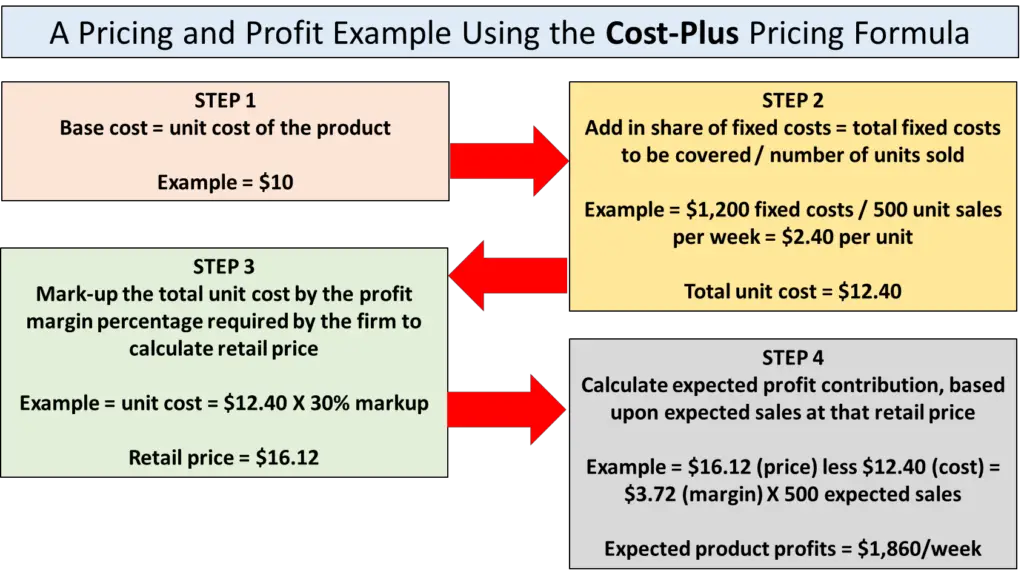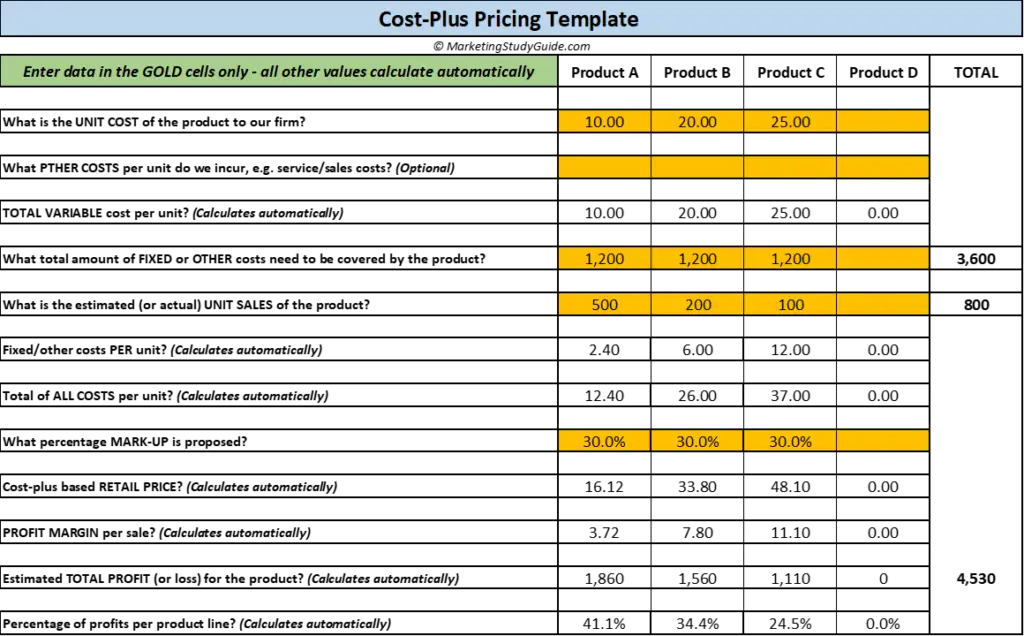# Cost-Plus Pricing Formula with Examples

In this article we look at the two formulas for calculating cost-plus pricing and provide several example calculations, plus there is a free cost-plus pricing Excel template available for download at the end of this post. Plus you can also review… More Cost-Plus Pricing Examples if you want more examples, or want to test yourself with the calculations.

• What is cost-plus pricing?
• What is the cost-plus pricing formula?
• Using the simple cost-plus pricing formula
• Using the cost-plus formula pricing with additional costs
• Cost-plus pricing formula examples
• Which is the best cost-plus pricing formula to use?

### What is Cost-plus pricing?

Cost-plus pricing is the easiest approach to pricing, as we are simply adding a percentage mark-up to the cost of the products that we are selling. As a result, it is also sometimes referred to as mark-up pricing.

As a simple example, let’s assume that we are a retailer, and we buy a product from our manufacturer for \$10. And let’s assume that we want a 50% mark-up on this cost price. That means that we will now sell this same product in our store for \$15, calculated as follows:

• Our retail price = \$10 unit cost PLUS a 50% mark-up = \$10 +\$5 = \$15

As you can see above, the terminology of cost-plus pricing comes from the above formula – where we take into account our cost and add/plus a profit margin. In the case above, our profit margin per product sale is \$5, as we have marked up the product’s cost by 50%.

#### What is the difference between cost-plus pricing and mark-up pricing?

There is no difference between cost-plus pricing and mark-up pricing. These two terms both refer to the same pricing method.

However, the term “mark-up” refers to the percentage that we use in the formula. In the above formula example, we used a mark-up of 50%.

Therefore, while there is no difference between the terms when describing the approach to pricing, it may refer to slightly different things depending upon the context. For instance, in the above example:

• Question: What pricing approach are we using? Answer: We used the cost-plus pricing method
• Question: What percentage mark-up did we use? = Answer: We used a 50% mark-up.

# What is the cost-plus pricing formula?

There are two approaches to calculating a retail price using the cost plus method.

The first approach is simplistic and applies a percentage markup to the unit cost of the product only. Whereas the second costing approach builds in additional costs including fixed costs and related variable costs.

Here are the two cost-plus pricing formulas:

1. Retail price = unit product cost X percentage markup
2. Retail price = (share of fixed costs/unit sales + related variable costs + unit product cost) X % percentage markup

Let’s work through both cost-plus formulas and then discuss which one is appropriate to use and when.

## An Example Using the Simple Cost-Plus Pricing Formula

Let’s assume that we are a retailer and that we buy three different products for our store, which we pay the following prices:

• Product 1 = \$10 per unit cost
• Product 2 = \$20 per unit cost
• Product 3 = \$25 per unit cost

And the manager/owner has decided that they want a 60% mark-up = which is their targeted gross profit margin per product sale.

Using the simple cost-plus formula, then the retailer would set their retail price for each product at:

• Product 1 = \$10 X 1.60 = \$16 retail price – giving a profit margin per unit of \$6
• Product 2 = \$20 X 1.60 = \$32 retail price – giving a profit margin per unit of \$12
• Product 3 = \$25 X 1.60 = \$40 retail price – giving a profit margin per unit of \$15

Calculating and Estimating Financial Outcomes

Once we know the profit margin per unit (as per the calculations above), we can then work out the gross product contribution for each product line by multiplying the unit margin by an estimate of (or actual) expected sales, as follows:

• Product 1 = \$6 profit margin X 500 unit sales/week = \$3,000 gross profit contribution
• Product 2 = \$12 profit margin X 200 unit sales/week= \$2,40= gross profit contribution
• Product 3 = \$15 profit margin X 100 unit sales/week = \$1,500 =gross profit contribution

As can be seen, these three products are expected to generate \$6,900 profit contribution per week for the retailer, based upon the sales volumes listed above and using a 60% mark-up.

However, this calculation does NOT take into account any other and fixed costs that also need to be covered by the retailer. That is why we generally need to use the following cost-plus formula, which includes relevant costs.

## An Example Using The Cost-Plus Pricing Formula with Additional Costs

Let’s now look at the second cost-plus formula, which builds in non-product costs into the calculation, which are typically fixed costs and other (non-product) variable costs.

For this calculation, let’s assume that this same retailer has the following cost structure:

• The rental cost of their store is \$1,000 per week (a fixed cost)
• Their staff costs are \$2,000 per week (a fixed cost)
• Their advertising costs are \$600 a week (a semi-variable cost, as it may change)
• This gives them a total fixed cost structure of \$3,600 per week
• Each of the three product lines are expected to generate sufficient profit to cover their equal share of fixed costs – that is, \$1,200 each
• And their unit cost structure is the same as the above example, which is:
• Product 1 = \$10 per unit cost
• Product 2 = \$20 per unit cost
• Product 3 = \$25 per unit cost

Please note for this formula, because we are building in fixed and potentially any other variable costs, it has the effect of increasing the base unit cost – we can use a LOWER percentage markup – and for the following example we will use a 30% markup.

Therefore, using the cost-plus formula, our retail price would be set at:

• Product 1 = \$10 unit cost + \$2.40 (\$1,200/500 = share of fixed costs) = \$12.40 TOTAL cost per unit X 30% mark-up = \$16.12 retail price (and \$3.72 margin)
• Product 2 = \$20 unit cost + \$6.00 (\$1,200/200 = share of fixed costs) = \$26 TOTAL cost per unit X 30% mark-up = \$33.80 retail price (and \$7.80 margin)
• Product 3 = \$25 unit cost + \$12 (\$1,200/100 = share of fixed costs) = \$37 TOTAL cost per unit X 30% mark-up = \$48.10 retail price (and \$11.10 margin)

Note on share of fixed costs – the calculation splits the firm’s total \$3,600 fixed costs evenly between the three products at \$1200 each, and then divides by expected unit sales to give a per unit cost. For example, for Product 1, \$2.40 of each sale needs to be allocated back to cover fixed costs.

Please note that the Product 1 retail price calculation, using the above cost-plus example is detailed below in the diagram:Calculating and Estimating Financial Outcomes

As we now have the retail price and the profit margin per unit, we can now multiple it by estimated sales to forecast overall profitability, as follows:

• Product 1 = \$3.72 profit margin X 500 unit sales = \$1.860 = net profit contribution
• Product 2 = \$7.80 profit margin X 200 unit sales = \$1,560 = net profit contribution
• Product 3 = \$11.10 profit margin X 100 unit sales = \$1,110 = net profit contribution

### Which is the Best Cost-Plus Formula to Use?

In most cases, the second cost-plus pricing formula with the approach (with the additional fixed costs included) is the BEST approach. The reason is that you can then be assured that the profitability from product sales will cover all other costs associated with the business.

If we use the simple cost-plus formula, without consideration of what fixed and other variable costs may need to be covered, it is indeed possible to price products TOO LOW and generate a loss for the business.

Let’s quickly compare the two profit outcomes from the two cost-plus formula approaches.

Using the simple cost-plus pricing formula method:

• Product 1 = \$3,000 = gross profit contribution
• Product 2 = \$2,400 = gross profit contribution
• Product 3 = = \$1,500 = gross profit contribution

And using the cost-plus pricing formula with additional costs method:

• Product 1 = \$1.860 = net profit contribution
• Product 2 = \$1,560 = net profit contribution
• Product 3 = \$1,110 = net profit contribution

In the first formula, you can see that the profit contribution is referred to as “gross” profit – which means that other costs have not been accounted for. Whereas, the second approach uses the word “net” profit which means that this is bottom-line profitability after costs have been recovered.

As a result, the second method gives you a very strong sense of what profits will be generated from the business after all costs. It is the best approach to cost-plus pricing and using the formula, because we have a true indication of the actual costs.

In this case, we are retailer, so staff and rental costs are critical to our business model and are part of the cost of providing the product to the end consumer. This is why this formula is generally considered superior.

Although the simple cost-plus formula generated a higher profit level (\$6,900 versus \$4,530), this simple approach has not considered the staff, rental, and marketing costs of \$3,600 per week. This means that the NET profit position is actually only \$3,300 (\$6,900 less \$3,600) after costs are considered.

Therefore, an additional risk with the simple cost-plus formula model is that we may overestimate profitability, as we have not considered all the costs involved in providing the product to the end consumer.

### When is the Simple Cost-plus Formula Appropriate to Use?

Having said that the second cost-plus formula is more appropriate and should be used in most cases, this is not to say that the simple cost-plus formula method does not suitable applications.

For example, if we are a large retailer running thousands of product lines, then using a simple cost-plus formula is probably going to be the best approach. This is because it will simplify the calculation.

For a large retailer, potentially with multiple stores and a large workforce, allocating share of fixed costs back to individual products or product lines can be quite cumbersome and potentially somewhat misleading if we get the allocation incorrect.

Therefore, if we are buying and selling thousands of unique products, then the simplest approach is to use the simple cost-plus formula – without reference to a share of fixed cost allocation. This means that it is very clear what the retail price will be of any product that comes into the store.

When using this approach, we need to make sure that OVERALL the combined profit more than adequately covers our fixed costs. This will give us financial reassurance, while also knowing that each product line has been marked up in price and therefore is profitable in its own right. That is, it provides a gross profit contribution.

You can either follow the steps above, or you can download the free Excel template for easily calculating retail prices using the cost-plus pricing formula. A great and easy to use tool for scenario testing of different price points and markups.